RS Aggarwal Solutions: Perimeter and Area of Plane Figures- 3

# RS Aggarwal Solutions: Perimeter and Area of Plane Figures- 3 - RS Aggarwal Solutions for Class 10 Mathematics

## Formative Assessment (Unit Test)

Q.1. In the given figure ABCD is quadrilateral in which ∠ABC = 90°, ∠BDC = 90°, AC = 17 cm, BC = 15 cm, BD = 12 cm and CD = 9 cm. The area of quad ABCD is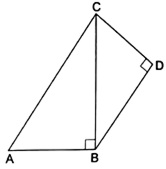(a) 102 cm2
(b) 114 cm2
(c) 95 cm2
(d) 57 cm
2

Given:
AC = 17 cm
BC = 15 cm
BD = 12 cm
CD = 9 cm.
∠ABC = 90°
∠BDC = 90°
In ∆ABC,
Using Pythagoras theorem,
AB2 + BC2 = AC2
⇒ AB2 = AC2- BC2
⇒ AB = √( AC2- BC2)
⇒ AB = √( 172- 152)
⇒ AB = √(289-225)
⇒ AB = √64
⇒ AB = 8 cm
Therefore,
Area of ∆ABC = 1/2 × AB × BC
= 1/2 × 8 × 15
= 60 cm2
And,
In ∆BDC,
Area of ∆BDC = 1/2 × BD × DC
= 1/2 × × 12 × 9
= 54 cm2
Therefore,
Area of quadrilateral ABCD = Area of ∆ABC + Area of ∆BDC
= 60 cm2 + 54 cm2
= 114 cm2

Q.2. In the given figure ABCD is a trapezium in which AB = 40 m, BC = 15 m, CD = 28 m, AD = 9 m and CE ⊥ AB. Area of trap. ABCD is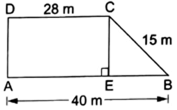(a) 306 m2
(b) 316 m2
(c) 296 m2
(d) 284 m2

ABCD is a trapezium in which AB = 40 m, BC = 15 m, CD = 28 m, AD = 9 m and CE ⊥ AB.
Area of trap. ABCD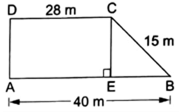AB = 40 m, BC = 15 m, AD = 9 m and CD = 28 m.
In trapezium ABCD,
Area of trapezium = 1/2 × sum of parallel sides × distance between them
= 1/2 × (28 + 40) × 9
= 1/2 × 68 × 9
= 306 m2

Q.3. The sides of a triangle are in the ratio 12 : 14 :25 and its perimeter is 25.5 cm. The largest side of the triangle is
(a) 7 cm
(b) 14 cm
(c) 12.5 cm
(d) 18 cm

Given: Ratio of Sides = 12: 14: 25
Perimeter = 25.5 cm
Let the sides be,
a = 12x cm
b = 14x cm
c = 25x cm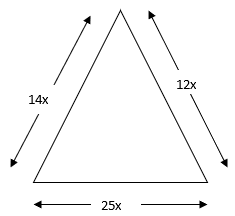We know that,
Perimeter of a triangle = a + b + c
⇒ 25.5 cm = 12x cm + 14x cm + 25x cm
⇒ 25.5 cm = 51x cm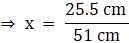⇒ x = 0. 5
Therefore,
a = 12x cm = 12 × 0.5 cm = 6 cm
b = 14x cm = 14 × 0.5 cm = 7 cm
c = 25x cm = 25 × 0.5 cm = 12.5 cm
Clearly largest side is c = 12.5 cm

Q.4. The parallel sides of a trapezium are 9.7 cm and 6.3 cm, and the distance between them is 6.5 cm. The area of the trapezium is
(a)104 cm2
(b) 78 cm2
(c) 52 cm2
(d) 65 cm2

Given:
Side 1 = 9.7 cm
Side 2 = 6.3 cm
Distance between sides = 6.5 cm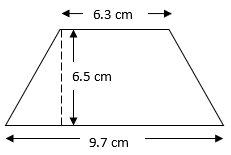Area of trapezium = 1/2 × sum of parallel sides × distance between them
= 1/2 × (9.7 + 6.3) × 6.5
= 1/2 × 16 × 6.5
= 52 cm2

Q.5. Find the area of an equilateral triangle having each side of length 10 cm. [Take √3 = 1.732.]

Given:
Side of an equilateral triangle = 10 cm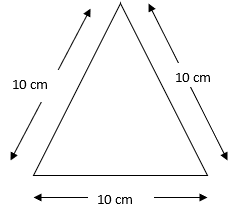Area of equilateral triangle =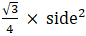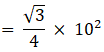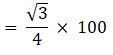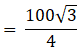= 25√3
= 25 × 1.732
= 43.3 cm2

Q.6. Find the area of an isosceles triangle each of whose equal side is 13 cm and whose base is 24 cm.

Given:
Side AB = Side AC = 13 cm
Base = 24 cm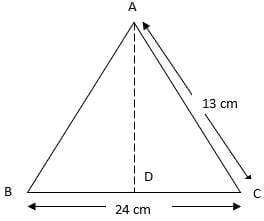DC = 12 cm
By Pythagoras theorem,
⇒ AD2 = 132 - 122
⇒ AD2 = 169 – 144 = 25
Now,
Area of triangle = 1/2 × base × height
= 1/2 × BC × AD
= 1/2 × 24 × 5
= 60 cm2

Q.7. The longer side of rectangular hall is 24 m and the length of its diagonal is 26 m. Find the area of the hall.

Given:
Length (l) = 24 m
Diagonal = 26 m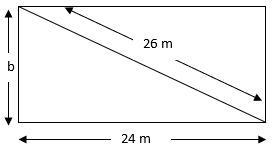We know that,
Base+ Perpendicular2 = Hypotenuse2
⇒ 242 + b2 = 262
⇒ b= 262 - 242
⇒ b2 = 676 - 576 = 100
⇒ b= 10 m
Area of rectangle = Length × Breadth
= 24 m × 10 m
= 240 m2

Q.8. The length of the diagonal of a square is 24 cm. Find its area.

Given:
Length of diagonal = 24 cm
Let the side of square = x cm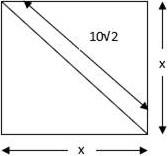We know that,
Hypotenuse2 = Base2 + Perpendicular2
⇒ 242 = x2 + x2
⇒ 576 = 2x2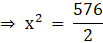⇒ x= 288
⇒ x = 12√2 cm
Now,
Area of a square = side2
= (12√2 cm)2
= 288 cm2

Q.9. Find the area of a rhombus whose diagonal are 48 cm and 20 cm long.

Given:
Length of diagonal 1 (d1) = 48 cm
Length of diagonal 2 (d2) = 20 cm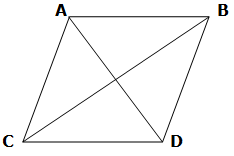Area of rhombus = 1/2 × d1 × d2
= 1/2 × 48 cm × 20 cm
= 480 cm2

Q.10. Find the area of a triangle whose sides are 42 cm, 34 cm and 20 cm.

Given: Side 1 = a (let) = 42 cm
Side 2 = b (let) = 34 cm
Side 3 = c (let) = 20 cm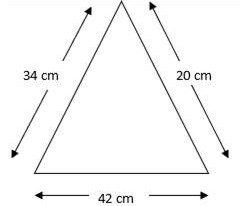We know that,
Area of a scalene triangle = √(s(s-a)(s-b)(s-c))
Where,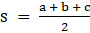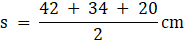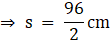⇒ s = 48 cm
Now,
Area of a scalene triangle = √(48cm × (48-42)cm × (48-34)cm × (48-20)cm)
= √(48cm × 6cm × 14cm × 28cm)
= √112896 cm2
= 336 cm2

Q.11. A lawn is in the form of a rectangle whose sides are in the ratio 5 : 3 and its area is 3375 m2. Find the cost of fencing the lawn at RS. 20 per metre.

Given: Cost of fencing lawn = Rs 20 per metre.
Area of lawn = 3375 m2
Let,
Length = 5x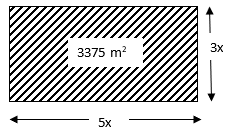We know that,
Area of lawn = Length × Breadth
⇒ 3375 m2 = 5x × 3x
⇒ 3375 m2 = 15x2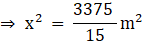⇒ x2 = 225 m2
⇒ x = 15 m
Therefore,
Length = 5x = 5 × 15 = 75 m
Breadth = 3x = 3 × 15 = 45 m
Now,
Perimeter of lawn = 2(length + breadth)
= 2(75 m + 45 m)
= 2 × 120 m
= 240 m
Hence,
Cost of Fencing = 240 m × Rs 20 per meter
= Rs 4800

Q.12. Find the area of a rhombus each side of which measurers 20 cm and one of whose diagonals is 24 cm.

Given:
Length of diagonal 1 (d1) = 24 cm
Side = 20 cm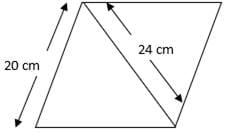Let, Length of diagonal 2 be d2
We know that,
Side of rhombus = 1/2 × √(d12 + d22 )
⇒ 20 = 1/2 × √(242 + d22 )
⇒ 20 × 2 = √(576 + d22 )
⇒ 40 = √(576 + d22)
Squaring both sides,
⇒ 1600 = 576 + d22
⇒ d22 = 1600-576
⇒ d22 = 1024
⇒ d2 = 32 cm
Now,
Area of rhombus= 1/2 × d1 × d2
= 1/2 × 24 × 32
= 384 cm2

Q.13. Find the area of a trapezium whose parallel sides are 11 cm and 25 cm long and non-parallel sides are 15 cm and 13 cm.

Given:
AB (say) = 11 cm
DC (say) = 25 cm
BC (say) = 13 cm
Draw AE ∥ BC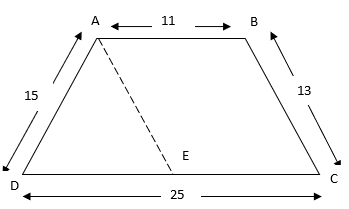Now the trapezium is divided into a triangle ADE and a parallelogram AECB.
Since, AECB is a parallelogram
Therefore, AE = BC = 13 cm
And, AB = EC
DE = DC – EC( = AB) = 25 – 11 = 14 cm
Now,
We know that,
Area of a scalene triangle (∆AED) = √(s(s-AE)(s-ED)(s-AD))
Where,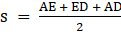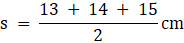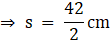⇒ s = 21 cm
Now,
Area of a scalene triangle = √(21cm × (21-13)cm × (21-14)cm × (21-15)cm)
= √(21cm × 8cm × 7cm × 6cm)
= √7056 cm2
= 84 cm2
Also,
Area of a triangle = 1/2 × base × height
⇒ 84 = 1/2 × 14 × height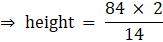⇒ height = 12 cm
Now,
Area of a parallelogram = base × height
= 11 cm × 12 cm
= 132 cm2
Now,
Area of Trapezium ABCD = Area of ∆ADE + Area of a parallelogram ABCE
= 84 cm2 + 132 cm2
= 216 cm2

Q.14. The adjacent sides of a llgm ABCD measure 34 cm and 20 cm and the diagonal AC is 42 cm long. Find the area of the ll gm.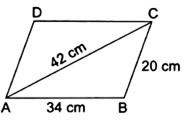Given:
AB = 34 cm
BC = 20 cm
AC = 42 cm
The diagonal of a parallelogram divides it into two equal triangles.
Therefore,
Area of ABCD = 2 × Area of ∆ABC
Now,
We know that,
Area of a scalene triangle = √(s(s-AC)(s-AB)(s-BC))
Where,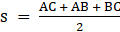⇒ s = 48cm
Now,
Area of a scalene triangle = √(48cm × (48-42)cm × (48-34)cm × (48-20)cm)
= √(48cm × 6cm × 14cm × 28cm)
= √112896 cm2
= 336 cm2
Therefore,
Area of ABCD = 2 × 336 cm2
= 672 cm2

Q.15. The cost of fencing a square lawn at RS. 14 per metre is RS. 2800. Find the cost of mowing the lawn at RS. 54 per 100m2.

Given:
Rate = RS. 14 per metre
Total Cost = RS. 2800
Rate of mowing = RS. 54 per 100 m2
Let the side of square field be s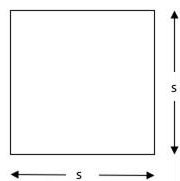Now,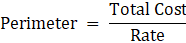= 2800/14
= 200 m
Perimeter = 4 × side
⇒ 200 m = 4 × s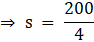⇒ s = 50 m
Now,
Area = side2
= (50 m) 2
= 2500 m2
Therefore,
Cost of mowing 100 m2 = Rs 54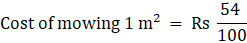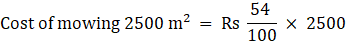= Rs 1350

Q.16. Find the area of quad. ABCD in which AB = 42 cm, BC = 21 cm, CD = 29 cm, DA = 34 cm and diag. BD = 20 cm.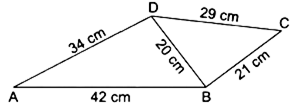Given:
DB = 20 cm
AB = 42 cm
CD = 29 cm
CB = 21 cm
In ∆ABD(scalene),
Area of a scalene triangle = √(s(s-AB)(s-BD)(s-AD))
Where,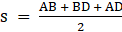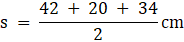⇒ s = 48 cm
Now,
Area of a scalene triangle = √(48cm × (48-42)cm × (48-20)cm × (48-34)cm)
= √(48 cm × 6 cm × 28 cm × 14 cm)
= √112896 cm2
= 336 cm2
Similarly,
In ∆BCD (scalene),
Area of a scalene triangle = √(s(s-BC)(s-CD)(s-BD))
Where,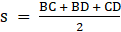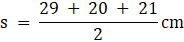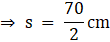⇒ s = 35 cm
Now,
Area of a scalene triangle = √(35 cm × (35-29)cm × (35-20)cm × (35-21)cm)
= √(35 cm × 6 cm × 15 cm × 14 cm)
= √44100 cm2
= 210 cm2
Now,
Area of quadrilateral ABCD = Area of ∆ABD + Area of ∆BCD
= 336 cm2 + 210 cm2
= 546 cm2

Q.17. A parallelogram and a rhombus are equal in area. The diagonals of the rhombus measure 120 m and 44 m. If one if the sides of the ll gm is 66 m long, find its corresponding altitude.

Given:
Diagonal 1 (d1) of rhombus = 120 m
Diagonal 2 (d2) of rhombus = 44 m
Side of parallelogram = 66 m
Area of rhombus = 1/2 × d1 × d2
= 1/2 × 120 m × 44 m
= 2640 m2
Now,
Area of parallelogram = Base × Height
⇒ 2640 m2 = 66 m × Height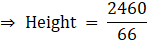⇒ Height = 40 m

Q.18. The diagonals of a rhombus are 48 cm and 20 cm long. Find the perimeter of the rhombus.

Given:
Length of diagonal 1 (d1) = 48cm
Length of diagonal 2 (d2) = 20 cmSide of rhombus = 1/2 × √(d12 + d22 )
= 1/2 × √(482 + 202 )
= 1/2 × √(2304 + 400)
= 1/2 × √2704
= 1/2 × 52
= 26 cm
Therefore,
Perimeter of rhombus = 4 × Side of rhombus
= 4 × 26 cm
= 104 cm

Q.19. The adjacent sides of a parallelogram are 36 cm and 27 cm in length. If the distance between the shorter sides is 12 cm, find the distance between the longer sides.

Given:
Longer side = 36 cm
Shorter side = 27 cm
Distance between Shorter sides = 12 cm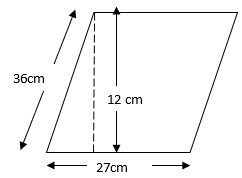Let, Distance between Longer sides = x cm
Now,
Area of parallelogram = Shorter Side × Distance between Longer sides
= 27 cm × 12 cm
= 324 cm2
Also,
Area of parallelogram = Longer side × Distance between Longer sides
⇒ 324 cm2 = 36 cm × x cm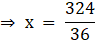⇒ x = 9 cm
Hence,
Distance between Shorter sides = 9 cm

Q.20. In a four sided field, the length of the longer diagonal is 128 m. The lengths of perpendiculars from the opposite vertices upon this diagonal are 22.7 m and 17.3 m. Find the area of the field.

Given:
BD = 128 m
CF = 22.7 m
AE = 17.3 m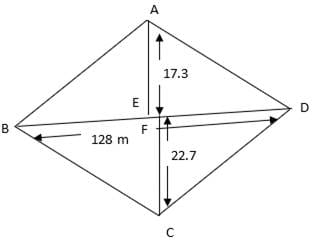Now,
In ∆ABD,
Area of a triangle = 1/2 × base × height
= 1/2 × BD × AE
= 1/2 × 128 × 17.3
= 1107.2 m2
Similarly,
In ∆CBD,
Area of a triangle = 1/2 × base × height
= 1/2 × BD × FC
= 1/2 × 128 × 22.7
= 1452.8 m2
Now,
Area of field = ∆ABD + ∆CBD
= 1107.2 m2 + 1452.8 m2
= 2560 m2

The document RS Aggarwal Solutions: Perimeter and Area of Plane Figures- 3 | RS Aggarwal Solutions for Class 10 Mathematics is a part of the Class 10 Course RS Aggarwal Solutions for Class 10 Mathematics.
All you need of Class 10 at this link: Class 10

53 docs|15 tests

## RS Aggarwal Solutions for Class 10 Mathematics

53 docs|15 testsExplore Courses for Class 10 examSignup to see your scores go up within 7 days! Learn & Practice with 1000+ FREE Notes, Videos & Tests.
10M+ students study on EduRev
Track your progress, build streaks, highlight & save important lessons and more!
Related Searches

,

,

,

,

,

,

,

,

,

,

,

,

,

,

,

,

,

,

,

,

,

;